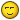# Ellipse drawing algorithm

## Recommended Posts

Hi!

I'm trying to script an ellipse drawing algorithm in AutoIT for learning purposes.
I use an edit control to "draw" the ellipse with characters.

The code i have works kinda good but i would like to see if others around here know a better algorithm to do it..
I would like to be able to give the x, y and width, height arguments to the function and not have to start at the middle point and draw the ellipse around it.

side note, the ellipse glitches when it has to be 2 pixels high too..

```func ellipsePlotPoints (\$xc,\$yc, \$x,\$y)

pixel (\$xc + \$x, \$yc + \$y)
pixel (\$xc - \$x, \$yc + \$y)
pixel (\$xc + \$x, \$yc - \$y)
pixel (\$xc - \$x, \$yc - \$y)

endfunc

func ellipse(\$xc,\$yc, \$a,\$b)

local \$a2 = \$a * \$a
local \$b2 = \$b * \$b
local \$twoa2 = 2 * \$a2
local \$twob2 = 2 * \$b2
local \$p
local \$x = 0
local \$y = \$b
local \$px = 0
local \$py = \$twoa2 * \$y

; Plot the initial point in each quadrant.
ellipsePlotPoints (\$xc,\$yc, \$x,\$y)

; Region 1
\$p = int(\$b2 - (\$a2 * \$b) + (0.25 * \$a2))
while (\$px < \$py)
\$x+=1
\$px += \$twob2
if (\$p < 0) then
\$p += \$b2 + \$px
else
\$y-=1
\$py -= \$twoa2
\$p += \$b2 + \$px - \$py
endif
ellipsePlotPoints (\$xc,\$yc, \$x,\$y)
wend

; Region 2
\$p = int (\$b2 * (\$x+0.5) * (\$x+0.5) + \$a2 * (\$y-1) * (\$y-1) - \$a2 * \$b2)
while (\$y > 0)
\$y-=1
\$py -= \$twoa2
if (\$p > 0) then
\$p += \$a2 - \$py
else
\$x+=1
\$px += \$twob2
\$p += \$a2 - \$py + \$px
endif
ellipsePlotPoints (\$xc,\$yc, \$x,\$y)
wend

endfunc```

##### Share on other sites

3 minutes ago, junkew said:

don't need a function that hides the actual code that draws the ellipsei need the code itself that puts the pixels where they are supposed to be

##### Share on other sites

here (https://www.autoitscript.com/forum/topic/181014-a-naive-question/?do=findComment&comment=1299979) you can find a simple implementation of the Bresenham's Algorithm, translated in AutoIt, to plot lines, circles, ellipses and Bézier curves plotted pixel by pixel (or character by character on a text box as well if you prefer)

Edited by ChimpChimp

small minds discuss people average minds discuss events great minds discuss ideas.... and use AutoIt....

##### Share on other sites

Chimp, Thanks so much, i'm gonna look in to that!
haha "a naive question"##### Share on other sites

Would be nice to know how to fill the ellipse too btw[edit:] if you draw an ellipse with your algorithm (w = 9, h = 4) then there are some pixels drawn twice..

Edited by TheAutomator

## Create an account

Register a new account

• ### Similar Content

• #### Detect pixel changes on specific screen area?

By kellariluukku,

• 13 replies
• 3,746 views
• #### AES-256-GCM Algorithm

By Colduction,

• 5 replies
• 1,505 views
• #### Drawing Script Plus

By v120,

• 2 replies
• 12,903 views
• #### Extracting color-values of image along a user specified line

By dejhost,

• 19 replies
• 3,452 views
• #### Line That Follows Cursor

• 0 replies
• 1,848 views
×

• Wiki

• Back

• #### Beta

• Git
• FAQ
×
• Create New...